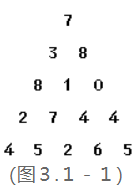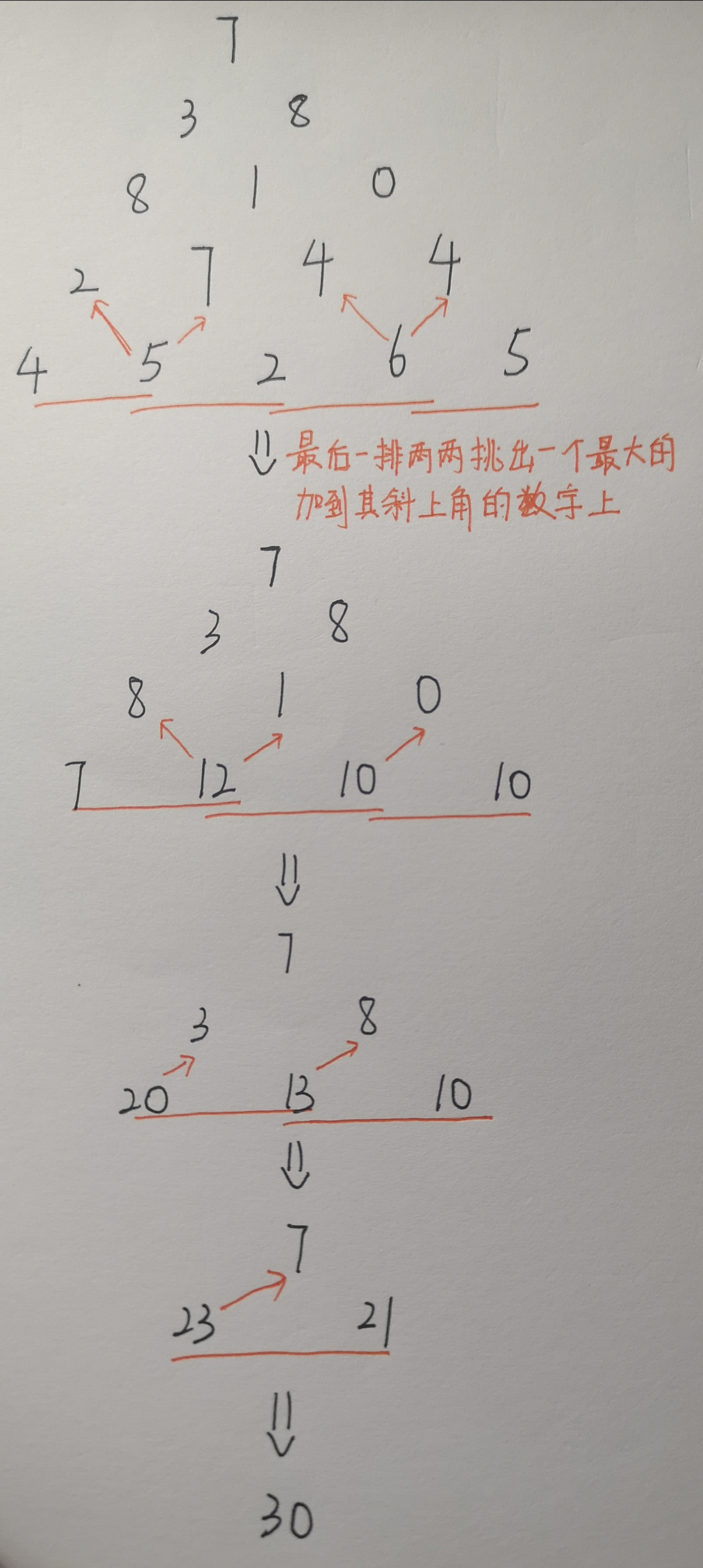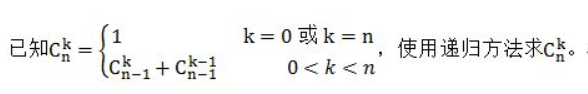# 练习Day——6

## 寂寞的数

33, 39, 51, 57, 69, 84, 96, 111, 114, 120, 123, 129, 141, …

n< =10000

40

1
3
5
7
9
20
31

import java.util.Scanner;

//寂寞的数
public class Main {
public static void main(String[]args) {
Scanner scan=new Scanner(System.in);
int n=scan.nextInt();

int num[]=new int[n];
boolean res[]=new boolean[n];//用来记录这个数是否是寂寞的数
for(int i=0;i<n;i++) {
res[i]=false;
}

//将非寂寞的数标记为true
for(int i=1;i<n;i++) {
num[i]=i;
int tmp=fun(num[i]);
if(tmp>=n)//如果这个非寂寞的数超过了n,则计算下一个
continue;
else//如果这个非寂寞的数在1~n-1的范围内，就标记为true
res[tmp]=true;
}
//输出所有false标记的数
for(int i=1;i<n;i++) {
if(res[i]==false) {
System.out.println(num[i]);
}
}
}

private static int fun(int i) {
int index=i;
while(i>0) {
index+=i%10;
i/=10;
}
return index;
}

}



## 未名湖边的烦恼

3 2

5

import java.util.Scanner;

//未名湖边的烦恼
public class Main {
public static void main(String[]args) {
Scanner scan=new Scanner(System.in);
int m=scan.nextInt();//还鞋
int n=scan.nextInt();//租鞋

System.out.println(fun(m,n));

}

private static int fun(int m, int n) {
if(n>m)//如果租鞋的人比还鞋的人多，那么一定会出现无鞋可租的情况，排法为0
return 0;
else if(n==0)//如果没有租鞋的人，那么就只有一种排法
return 1;
else//排了一个还鞋的人，剩下的人再重新排队 和 排了一个租鞋的人，剩下的人再重新排队
return fun(m-1,n)+fun(m,n-1);
}

}



## 数字三角形（ALGO-124）

（图３.１－１）示出了一个数字三角形。 请编一个程序计算从顶至底的某处的一条路径，使该路径所经过的数字的总和最大。
●每一步可沿左斜线向下或右斜线向下走；
●1＜三角形行数≤100；
●三角形中的数字为整数0，1，…99；5
7
3 8
8 1 0
2 7 4 4
4 5 2 6 5

30import java.util.Scanner;

//数字三角形
public class Main {
public static void main(String[]args) {
Scanner scan=new Scanner(System.in);
int n=scan.nextInt();//行数

int num[][]=new int;
for(int i=0;i<n;i++) {
for(int j=0;j<=i;j++) {
num[i][j]=scan.nextInt();
}
}

for(int i=n-2;i>=0;i--) {
for(int j=0;j<=i;j++) {
num[i][j]+=Math.max(num[i+1][j], num[i+1][j+1]);
}
}

System.out.println(num);
}
}


## 6-1 递归求二项式系数值3 10

120

import java.util.Scanner;

//6-1 递归求二项式系数值
public class Main {
public static void main(String[]args) {
Scanner scan=new Scanner(System.in);
int k,n;
k=scan.nextInt();
n=scan.nextInt();

System.out.println(C(k,n));
}

private static int C(int k, int n) {
if(k==0 || k==n)
return 1;
else
return C(k,n-1)+C(k-1,n-1);
}

}


## 最小乘积(基本型)

(-5) * 4 + 3 * (-2) + 1 * 1 = -25

n<=8,T<=1000

2
3
1 3 -5
-2 4 1
5
1 2 3 4 5
1 0 1 0 1

-25
6

import java.util.Scanner;

//最小乘积(基本型)
public class Main {
public static void main(String[]args) {
Scanner scan=new Scanner(System.in);
int sum=scan.nextInt();//测试数据组数
int res;//记录最小乘积

while(sum>0) {
res=0;
int n=scan.nextInt();
int num1[]=new int[n];
int num2[]=new int[n];

for(int i=0;i<n;i++)
num1[i]=scan.nextInt();

for(int i=0;i<n;i++)
num2[i]=scan.nextInt();

sort1(num1);//从大到小排列
sort2(num2);//从小到大排列

for(int i=0;i<n;i++) {
res=res+num1[i]*num2[i];
}

System.out.println(res);
sum--;
}
}

private static void sort1(int[] num) {
for(int i=0;i<num.length-1;i++) {
for(int j=i;j<num.length;j++) {
if(num[i]<num[j]) {
int tmp=num[i];
num[i]=num[j];
num[j]=tmp;
}
}
}

}

private static void sort2(int[] num) {
for(int i=0;i<num.length-1;i++) {
for(int j=i;j<num.length;j++) {
if(num[i]>num[j]) {
int tmp=num[i];
num[i]=num[j];
num[j]=tmp;
}
}
}

}

}


02-241272
02-237202-16118
10-1541
10-1443
08-1340
07-15353
02-18111
02-2667
02-17235